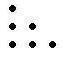# Patterns and Number Sequence: Fill in the Gaps

In this worksheet, students try to spot number patterns in well-known sequences and add missing numbers.Key stage:  KS 2

Curriculum topic:   Maths and Numerical Reasoning

Curriculum subtopic:   Sequences

Difficulty level:### QUESTION 1 of 10

In this worksheet, we will learn to define a pattern by counting dots and then describe the sequence.

For example -

Question -

Count the dots and write the numbers.
Describe the sequence. Write the next two numbers.

To describe the sequence you need to look at the difference between the numbers. For example it might increase by three each time.1
 3
 5
 7
 9
 11

These are odd numbers.

Count the dots and select the numbers that correspond to the next two boxes.

Also try and describe what type of sequence it is on a separate piece of paper.1

9

11

36

26

48

25

32

Count the dots and tick the numbers that correspond to the next two numbers in the sequence.
Describe the sequence. Write the next two numbers.16

8

5

15

20

21

• Question 1

Count the dots and select the numbers that correspond to the next two boxes.

Also try and describe what type of sequence it is on a separate piece of paper.36
25
EDDIE SAYS
These are square numbers.
• Question 2

Count the dots and tick the numbers that correspond to the next two numbers in the sequence.
Describe the sequence. Write the next two numbers.15
21
EDDIE SAYS
These are triangle numbers.
---- OR ----

Sign up for a £1 trial so you can track and measure your child's progress on this activity.

### What is EdPlace?

We're your National Curriculum aligned online education content provider helping each child succeed in English, maths and science from year 1 to GCSE. With an EdPlace account you’ll be able to track and measure progress, helping each child achieve their best. We build confidence and attainment by personalising each child’s learning at a level that suits them.

Get started